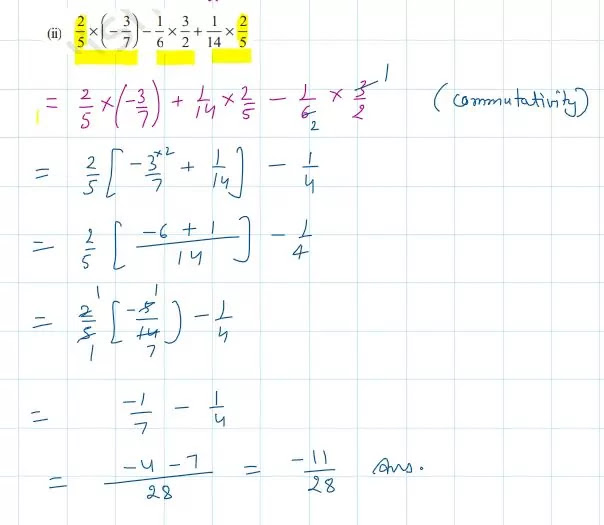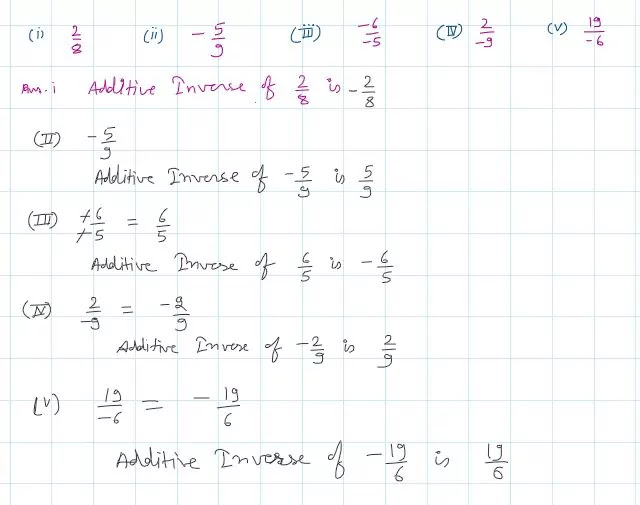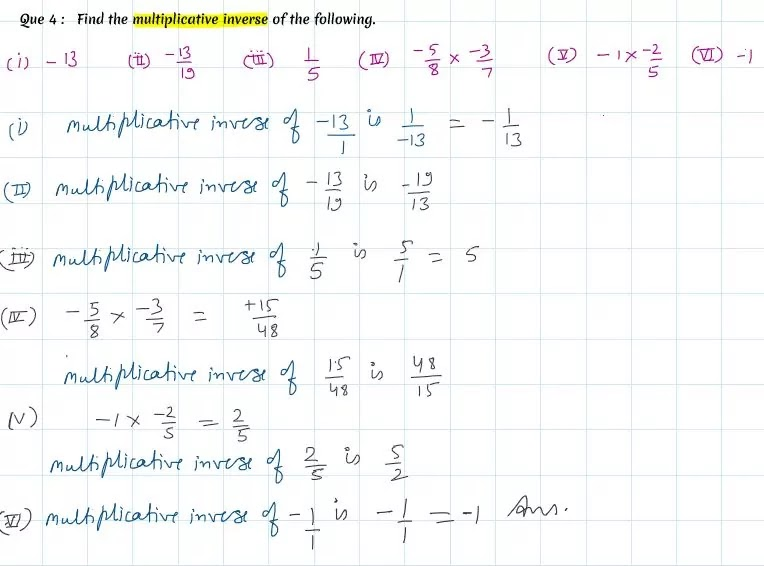Home » CBSE Class 8 Maths Chapter 1 Rational Numbers

# Chapter 1 Rational Numbers

NCERT CBSE Solutions Class 8 Maths Chapter 1 Rational Numbers

Class 8th Maths Chapter 1 Rational Number Ex 1.1 Que 1

Ex 1.1 class 8 question 1 Using appropriate properties find.
(i) – 2/3 × 3/5 + 5/2−3/5 × 1/6 – 2/3 × 3/5 + 5/2−3/5 × 1/6Class 8th Maths Chapter 1 Rational Number Ex 1.1 Que 1 (i)

Ex 1.1 class 8 question 1 Using appropriate properties find.
(ii) 2/5 x (-3/7) – 1/6 x 3/2 + 1/14 x 2/5.8 Maths Chapter 1 Rational Number Ex 1.1 Que 1 (ii)

Ex 1.1 class 8 question 2 : Write the additive inverse of each of the following
(i) 2/8 (ii) -5/9 (iii) -6/-5 (iv) 2/-9 (v) 19/-6Class 8th Math Chapter 1 Rational Number Ex 1.1 Question 2 (ii)

NCERT Class 8th Maths All Chapter Solution

NCERT Solution for Class 8

NCERT Solutions for Class 9

NCERT Solution for class 10

Ex 1.1 class 8 question 3 Verify that – (– x) = x for
(i) x=11/15 (ii) x=-13/17Ex 1.1 class 8 question 3

Ex 1.1 class 8 question 4 Find the multiplicative inverse of the following.Ex 1.1 class 8 question 4
Scroll to Top